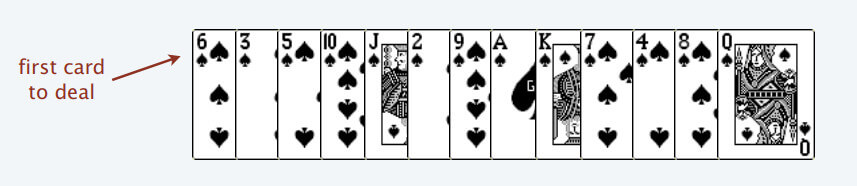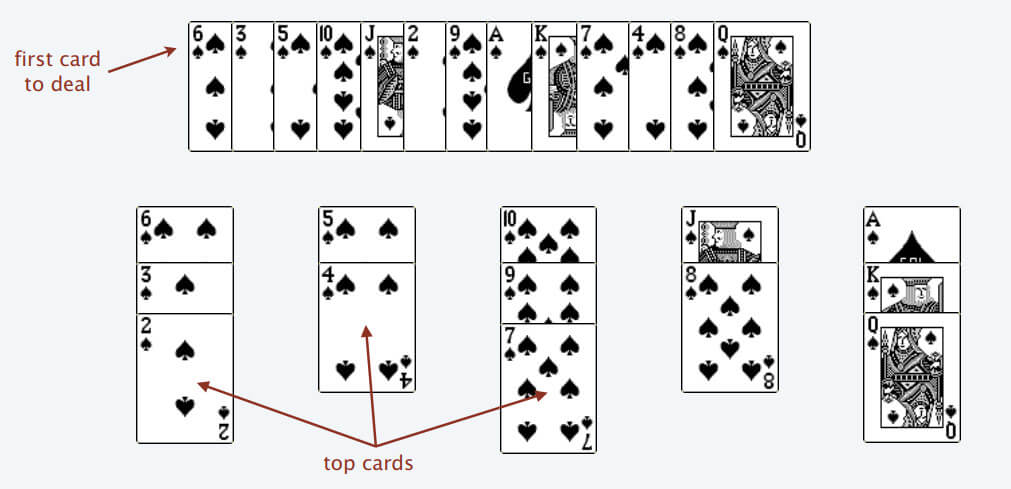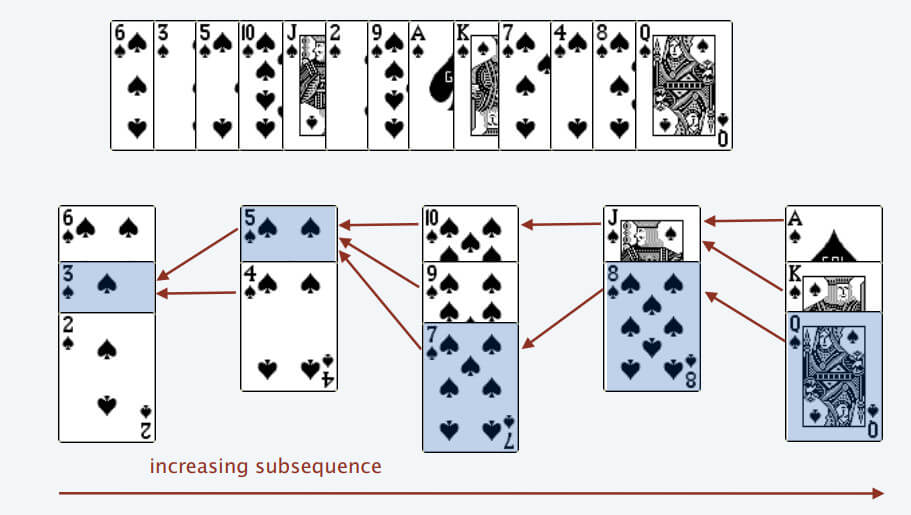# 🤖 leetcode 300. Longest Increasing Subsequence | medium | dynamic-programming | binary-search | javascript

## 🗒️ Problem

Longest Increasing Subsequence - LeetCode

``````Given an integer array nums,
return the length of the longest strictly increasing subsequence.

A subsequence is a sequence that can be derived from an array
by deleting some or no elements without changing the order of the remaining elements.
For example, [3,6,2,7] is a subsequence of the array [0,3,1,6,2,2,7].
``````
``````Input: nums = [10,9,2,5,3,7,101,18]
Output: 4
Explanation: The longest increasing subsequence is [2,3,7,101], therefore the length is 4.
``````

## ⬆️ Dynamic programming O(n^2)

• `dp[i]` : LIS (longest increasing subsequence) length ending in `nums[i]`.

### ⬆️ 🍀 Intuition

• If `nums[j] < nums[i]`
• we can get one larger subsequence than the previous one (`dp[j`)
``````for (let i = 0; i < n; i += 1) {
for (let j = 0; j < i; j += 1) {
if (nums[j] < nums[i]) dp[i] = Math.max(dp[i], dp[j] + 1);
}
}
``````

### ⬆️ 🔥 bottom solution

``````var lengthOfLIS = function (nums) {
const n = nums.length;

const dp = Array(n).fill(1);

for (let i = 0; i < n; i += 1) {
for (let j = 0; j < i; j += 1) {
if (nums[j] < nums[i]) dp[i] = Math.max(dp[i], dp[j] + 1);
}
}
return Math.max(...dp);
};
``````

I came to know by reading the Korean book 알라딘: 코딩 인터뷰를 위한 알고리즘 치트시트Same as the above card game rule.

• Cards are placed from the left to the bottom in the top row, and cards can be placed on top only if the value is smaller than the lower card.
• If the card selected in the upper row is larger than the card placed in the lower row (-> increasing sequence is created here), a new one is created on the right side of the lower row.
• If the value of the card selected in the upper row is too small to be placed in several rows in the lower row, it is placed at the far left.
• The number of columns created in the last lower row becomes the LIS length.

### 🔎 ✨ Algorithm

• Create an array (`piles[]`) representing the lines below.
• Choose a card from the far left on the top row and determine where it should be placed on the bottom row.
• At this time, use the binary search left to find the insertion point of the selected card.
• If there is a larger card (insertion point is < length), place it on the card at the corresponding location (overwrite the value of the corresponding index)
• If the insertion point is `length` because there is no larger card, increase the array size and insert it at the end.
• ...
• The length of the final array becomes LIS.
``````/**
* @param {number[]} nums
* @return {number}
*/
var lengthOfLIS = function (nums) {
const bisectLeft = (array, target) => {
const N = array.length;

let left = 0;
let right = N;

while (left < right) {
const mid = Math.floor((left + right) / 2);

if (array[mid] === target) {
right = mid;
} else if (array[mid] > target) {
right = mid;
} else if (array[mid] < target) {
left = mid + 1;
}
}
return left;
};

const N = nums.length;
const piles = [];

for (const num of nums) {
const index = bisectLeft(piles, num);

if (index === piles.length) piles.push(num);
else piles[index] = num;
}

return piles.length;
};
``````